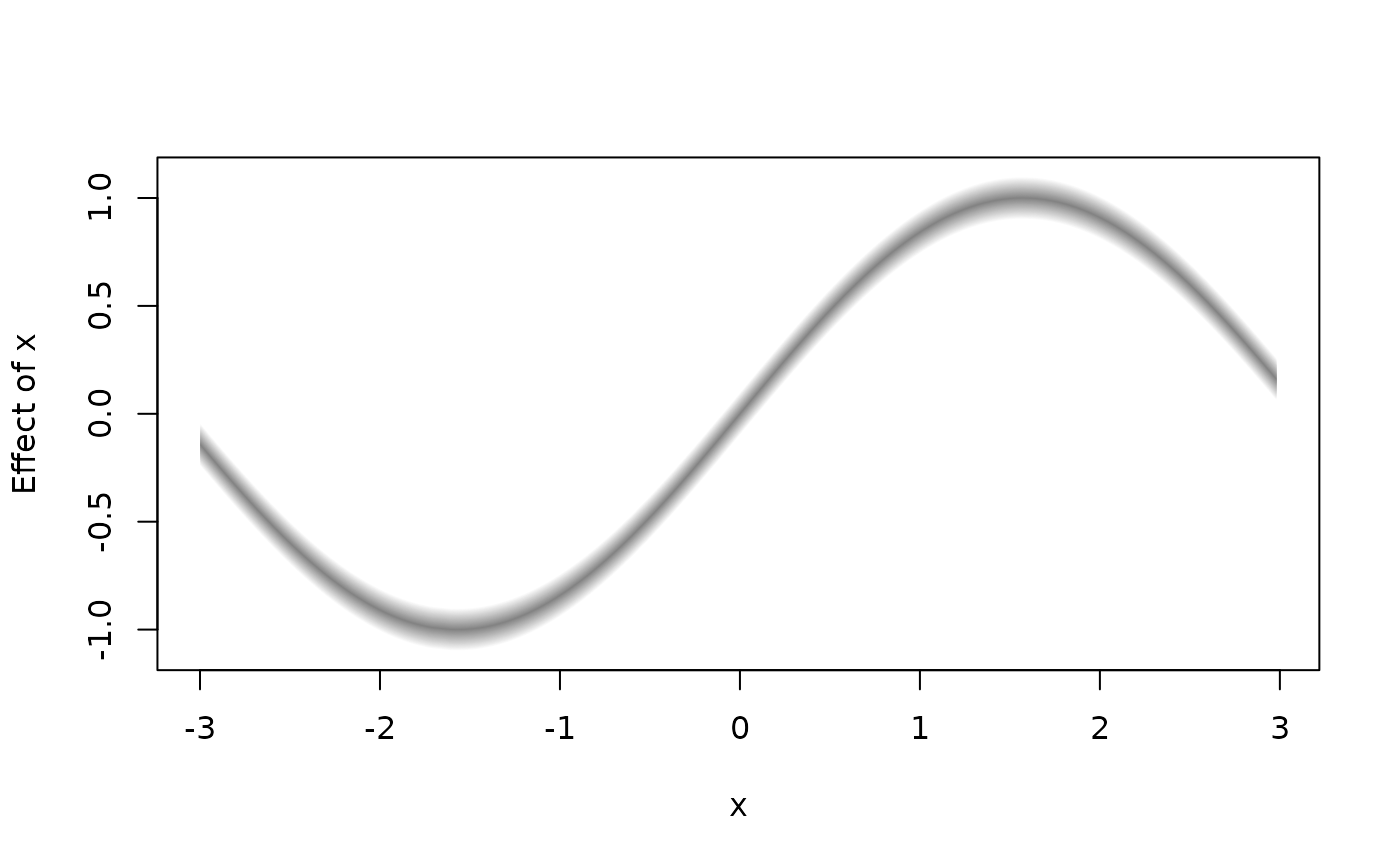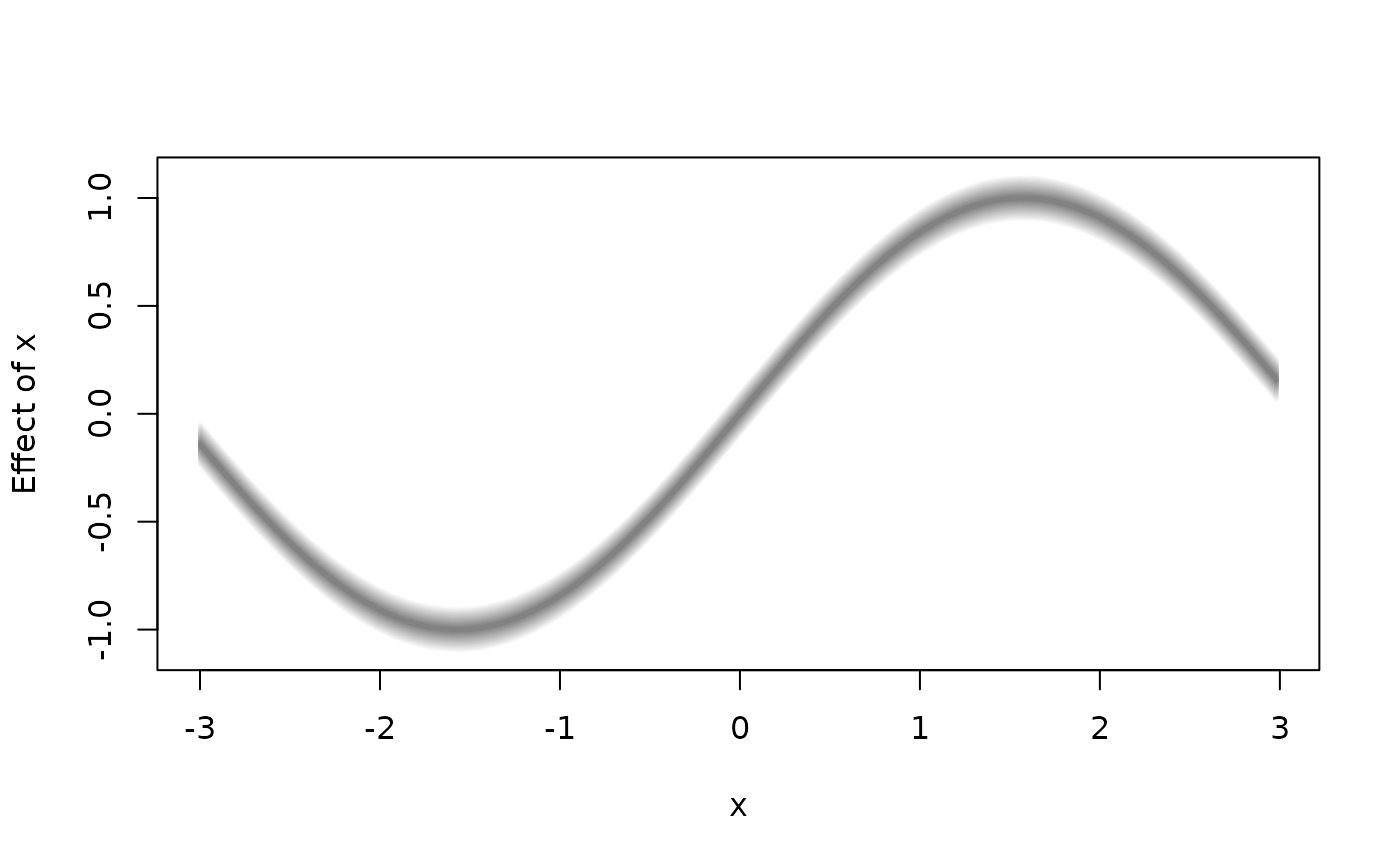Function to plot simple 2D graphics for univariate effects/functions.

plot2d(x, residuals = FALSE, rug = FALSE, jitter = TRUE,
col.residuals = NULL, col.lines = NULL, col.polygons = NULL,
col.rug = NULL, c.select = NULL, fill.select = NULL,
data = NULL, sep = "", month = NULL, year = NULL,
step = 12, shift = NULL, trans = NULL,
scheme = 2, s2.col = NULL, grid = 50, ...)

## Arguments

x

A matrix or data frame, containing the covariate for which the effect should be plotted in the first column and at least a second column containing the effect. Another possibility is to specify the plot via a formula, e.g. y ~ x, see the examples. x may also be a character file path to the data to be used for plotting.

residuals

If set to TRUE, residuals may also be plotted if available. Residuals must be supplied as an attribute named "residuals", which is a matrix or data frame where the first column is the covariate and the second column the residuals.

rug

Add a rug to the plot.

jitter

If set to TRUE a jittered rug plot is added.

col.residuals

The color of the partial residuals.

col.lines

The color of the lines.

col.polygons

Specify the background color of polygons, if x has at least 3 columns, i.e. column 2 and 3 can form one polygon.

col.rug

Specify the color of the rug representation.

c.select

Integer vector of maximum length of columns of x, selects the columns of the resulting data matrix that should be used for plotting. E.g. if x has 5 columns, then c.select = c(1, 2, 5) will select column 1, 2 and 5 for plotting. Note that first element of c.select should always be the column that holds the variable for the x-axis.

fill.select

Integer vector, select pairwise the columns of the resulting data matrix that should form one polygon with a certain background color specified in argument col. E.g. x has three columns, or is specified with formula f1 + f2 ~ x, then setting fill.select = c(0, 1, 1) will draw a polygon with f1 and f2 as boundaries. If x has five columns or the formula is e.g. f1 + f2 + f3 + f4 ~ x, then setting fill.select = c(0, 1, 1, 2, 2), the pairs f1, f2 and f3, f4 are selected to form two polygons.

data

If x is a formula, a data.frame or list. By default the variables are taken from environment(x): typically the environment from which plot2d is called. Note that data may also be a character file path to the data.

sep

The field separator character when x or data is a character, see function read.table.

month, year, step

Provide specific annotation for plotting estimation results for temporal variables. month and year define the minimum time point whereas step specifies the type of temporal data with step = 4, step = 2 and step = 1 corresponding to quarterly, half yearly and yearly data.

shift

Numeric constant to be added to the smooth before plotting.

trans

Function to be applied to the smooth before plotting, e.g., to transform the plot to the response scale.

scheme

Sets the plotting scheme for polygons, possible values are 1 and 2.

s2.col

The color for the second plotting scheme.

grid

Integer, specifies the number of polygons for the second plotting scheme.

...

Other graphical parameters, please see the details.

## Details

For 2D plots the following graphical parameters may be specified additionally:

• cex: Specify the size of partial residuals,

• lty: The line type for each column that is plotted, e.g. lty = c(1, 2),

• lwd: The line width for each column that is plotted, e.g. lwd = c(1, 2),

• poly.lty: The line type to be used for the polygons,

• poly.lwd: The line width to be used for the polygons,

• density angle, border: See polygon,

• ...: Other graphical parameters, see function plot.

plot3d, plotmap, plotblock, sliceplot.

## Examples

## Generate some data.
set.seed(111)
n <- 500
## Regressor.
d <- data.frame(x = runif(n, -3, 3))

## Response.
d$y <- with(d, 10 + sin(x) + rnorm(n, sd = 0.6)) if (FALSE) ## Estimate model. b <- bamlss(y ~ s(x), data = d) summary(b) #> Error in summary(b): object 'b' not found ## Plot estimated effect. plot(b) #> Error in h(simpleError(msg, call)): error in evaluating the argument 'x' in selecting a method for function 'plot': object 'b' not found plot(b, rug = FALSE) #> Error in h(simpleError(msg, call)): error in evaluating the argument 'x' in selecting a method for function 'plot': object 'b' not found ## Extract fitted values. f <- fitted(b, model = "mu", term = "s(x)") #> Error in fitted(b, model = "mu", term = "s(x)"): object 'b' not found f <- cbind(d["x"], f) #> Error in data.frame(..., check.names = FALSE): object 'f' not found ## Now use plot2d. plot2d(f) #> Error in plot2d(f): object 'f' not found plot2d(f, fill.select = c(0, 1, 0, 1)) #> Error in plot2d(f, fill.select = c(0, 1, 0, 1)): object 'f' not found plot2d(f, fill.select = c(0, 1, 0, 1), lty = c(2, 1, 2)) #> Error in plot2d(f, fill.select = c(0, 1, 0, 1), lty = c(2, 1, 2)): object 'f' not found plot2d(f, fill.select = c(0, 1, 0, 1), lty = c(2, 1, 2), scheme = 2) #> Error in plot2d(f, fill.select = c(0, 1, 0, 1), lty = c(2, 1, 2), scheme = 2): object 'f' not found ## Variations. plot2d(sin(x) ~ x, data = d) d$f <- with(d, sin(d$x)) plot2d(f ~ x, data = d)d$f1 <- with(d, f + 0.1)
d\$f2 <- with(d, f - 0.1)
plot2d(f1 + f2 ~ x, data = d)plot2d(f1 + f2 ~ x, data = d, fill.select = c(0, 1, 1), lty = 0)plot2d(f1 + f2 ~ x, data = d, fill.select = c(0, 1, 1), lty = 0,
density = 20, poly.lty = 1, poly.lwd = 2)plot2d(f1 + f + f2 ~ x, data = d, fill.select = c(0, 1, 0, 1),
lty = c(0, 1, 0), density = 20, poly.lty = 1, poly.lwd = 2)